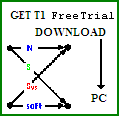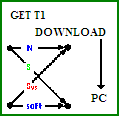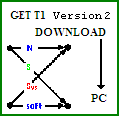# AdvDCSM Bit Error Rate (BER) Plot Generation Feature

NOTE: This section is Under-Edit if necessary: Construction began on December 15, 2012 and finished on December 15, 2012. This page has been revised on March 2 2022 so that the T1 Professional BER Plot could be viewed via a Download and Open of its PDF File.

This Bit Error Rate (BER)/Bit Error Probability (Pb) Plot Generation feature has been added to AdvDCSMT1DCSS : T1 [T1 Version 1, T1 Trial Version 1, and T1 Version 2 (T1 Professional)] so that the User can create a BER plot using an easy-to-use Natural Language Query and Visual Builders Input method.

A T1 BER Plot consists of three major components:
```1) BER (Pb) versus SNR [Eb/N0 (dB)] Axes, Axis Labels and Curves;2) Legend (Curve Description-Symbol Mapping);3) Figure Description.
```
In T1 Professional, one can create a BER Plot that consists of one (1) to six (6) BER curves where each curve can consist of three (3) to sixteen (16) data points where a data point is [Eb/N0 (dB), Pb].

In T1 Version 1, one can create a BER Plot that consists of one (1) to six (6) BER curves where each curve can consist of three (3) to sixteen (16) data points where a data point is [Eb/N0 (dB), Pb].

In T1 Trial Version 1, one can create a BER Plot that consists of one (1) to five (5) BER curves where each curve can consist of three (3) to sixteen (16) data points where a data point is [Eb/N0 (dB), Pb].

The BER Plot Generation Feature consists of two modes of operation:
```1) New Plot Generation; &2) Edit an existing BER Plot.
```
This feature will auto-scale the SNR (dB) axis values based on the range of the inputted Eb/N0 (dB) values of the BER plot data points.

The general approach to using this feature is as follows:

First, the User of T1 will run T1 to collect a BER plot's simulated Pb results (a data point of a BER curve is obtained from each simulation run); and

Second, the User then uses T1 to generate a BER plot by choosing the BER Plot Generation Feature (via the Design Menu) and then entering the appropriate responses to the Queries (choices and Plot data into T1 at the apt time as called for by T1).

A T1 Professional generated BER Plot example (Punctured Convolutional Coded M-ary Signaling over a Coherent Memoryless Channel with AWGN) can be viewed via a Download (click on the below DOWNLOAD LINK). This Plot (.PLT-BER file extension) was printed by T1 via a HP device; the printed plot was scanned by a HP device and saved as a Adobe pdf file.

Note that for a screen display of a BER Plot, each curve (& its data point symbols) is assigned an unique color. For a printed plot, each curve (& its data point symbols) is set to be black so that there is no fading of the curve due to the use of a certain color on a black and white printer.

```GET FREE TRIAL USE of T1, AdvDCSMT1DCSS by DOWNLOAD.
```BUY T1 (ADVDCSMT1DCSS software system tool)NOW.
```BUY T1 Version 2 (ADVDCSMT1DCSS Professional software system tool)NOW.